↑ 收起筛选 ↑

(1)若a＝2，b＝，求cosC的值；

(2)若sinAcos2＋sinB·cos2＝2sinC，且△ABC的面积S＝sinC，求a和b的值．

• 已知A，B，C为△ABC的三个内角；a，b，c分别为对边，向量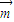=（2cosC-1，-2），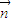=（cosC，cosC+1），若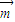，且a+b=10，则△ABC周长的最小值为（ ）
A．10-5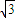B．10+5C．10-2D．10+2高三数学选择题中等难度题查看答案及解析

• 已知A，B，C为△ABC的三个内角；a，b，c分别为对边，向量=（2cosC-1，-2），=（cosC，cosC+1），若，且a+b=10，则△ABC周长的最小值为（ ）
A．10-5B．10+5C．10-2D．10+2高三数学选择题中等难度题查看答案及解析

• 已知△ABC的内角A，B，C的对边分别为a，b，c，其中c=2，又向量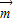=（1，cosC），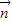=（cosC，1），=1．
（1）若A=45°，求a的值；
（2）若a+b=4，求△ABC的面积．

高三数学解答题中等难度题查看答案及解析

• 已知△ABC的内角A，B，C的对边分别为a，b，c，其中c=2，又向量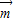=（1，cosC），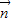=（cosC，1），=1．
（1）若A=45°，求a的值；
（2）若a+b=4，求△ABC的面积．

高三数学解答题中等难度题查看答案及解析

• 已知△ABC的内角A，B，C的对边分别为a，b，c，其中c=2，又向量=（1，cosC），=（cosC，1），=1．
（1）若A=45°，求a的值；
（2）若a+b=4，求△ABC的面积．

高三数学解答题中等难度题查看答案及解析

• 已知△ABC的内角A，B，C的对边分别为a，b，c，其中c=2，又向量=（1，cosC），=（cosC，1），=1．
（1）若A=45°，求a的值；
（2）若a+b=4，求△ABC的面积．

高三数学解答题中等难度题查看答案及解析

• 已知△ABC的内角A，B，C的对边分别为a，b，c，其中c=2，又向量=（1，cosC），=（cosC，1），=1．
（1）若A=45°，求a的值；
（2）若a+b=4，求△ABC的面积．

高三数学解答题中等难度题查看答案及解析

• 在锐角△ABC中，角A，B，C所对的边分别为a，b，c，已知向量=（2b-c，cosC），=（a，cosA），且（Ⅰ）求角A的大小；
（Ⅱ）求cosB+cosC的取值范围．

高三数学解答题中等难度题查看答案及解析

• 在△ABC中，∠A，∠B，∠C所对的边分别为a，b，c，则下列关系正确的是（ ）
A．cosC=a2+b2-c2
B．cosC=a2-b2+c2
C．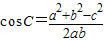D．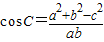高二数学选择题中等难度题查看答案及解析

• 在△ABC中，∠A，∠B，∠C所对的边分别为a，b，c，则下列关系正确的是（ ）
A．cosC=a2+b2-c2
B．cosC=a2-b2+c2
C．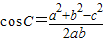D．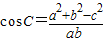高二数学选择题中等难度题查看答案及解析